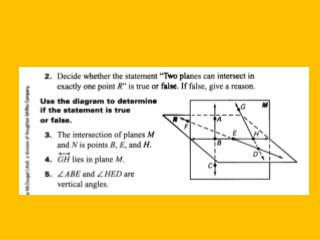DownloadDownload PresentationPoints, Lines, and Planes

# Points, Lines, and Planes

Download Presentation## Points, Lines, and Planes

- - - - - - - - - - - - - - - - - - - - - - - - - - - E N D - - - - - - - - - - - - - - - - - - - - - - - - - - -
##### Presentation Transcript

1. Points, Lines, and Planes Section 1.2 Segments and Congruence Section 1.3 Use Midpoint and Distance Formulas

2. Ruler Postulate • The points on a line can be matched one to one with the real numbers. • There are an infinite number of points on a line and an infinite number of real numbers. • The real number that corresponds to the point is the coordinate of the point.

3. AB The distance between point A and point B. The length of AB. A B . . AB = 12 12

4. AB means “the distance between point A and Point B”. (number) • AB means “line AB”. (figure) • AB means “segment AB”. (figure) • AB means “ray AB”. (figure)

5. Distance Formulas Number Line Coordinate Plane Distance Formula • Absolute value of the difference between the coordinates . A . B √

6. You can only use the word “between” if all three points are collinear. . . . A B C B is between A and C . E . . D F E is not between D and F

7. Segment Addition Postulate If B is between A and C, then AB + BC = AC. If AB + BC = AC, then B is between A and C. . . . 12 5 C A B 17

8. Congruent Segments Line segments that are the same length. AB = CD The lengths are equal. The Segments are congruent. . . . . A B C D

9. Midpoint The point that divides the segment into two congruent segments. A segment has exactly one midpoint. . . . A M B M is the midpoint of AB.

10. Segment Bisector • A point, ray, line, line segment , or plane that intersects a segment at its midpoint. • A segment can have an infinite number of bisectors. . • . • .

11. Midpoint Formula Number Line Coordinate Plane The coordinates of the midpoint of a segment whose endpoints have coordinates a and b is